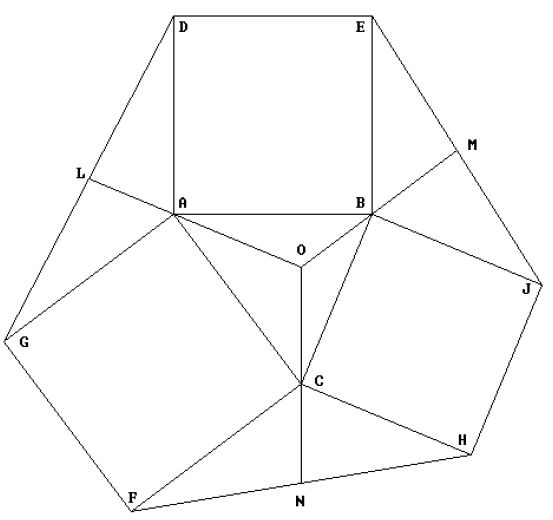Online JudgeProblem SetAuthorsOnline ContestsUser
Web Board
F.A.Qs
Statistical Charts
Problems
Submit Problem
Online Status
Prob.ID:
Register
Authors ranklist
Current Contest
Past Contests
Scheduled Contests
Award Contest
Register
Language:
EXOCENTER OF A TRIANGLE
 Time Limit: 1000MS Memory Limit: 10000K Total Submissions: 3850 Accepted: 1541

Description

Given a triangle ABC, the Extriangles of ABC are constructed as follows:
On each side of ABC, construct a square (ABDE, BCHJ and ACFG in the figure below).
Connect adjacent square corners to form the three Extriangles (AGD, BEJ and CFH in the figure).
The Exomedians of ABC are the medians of the Extriangles, which pass through vertices of the original triangle,extended into the original triangle (LAO, MBO and NCO in the figure. As the figure indicates, the three Exomedians intersect at a common point called the Exocenter (point O in the figure).
This problem is to write a program to compute the Exocenters of triangles.Input

The first line of the input consists of a positive integer n, which is the number of datasets that follow. Each dataset consists of 3 lines; each line contains two floating point values which represent the (two -dimensional) coordinate of one vertex of a triangle. So, there are total of (n*3) + 1 lines of input. Note: All input triangles wi ll be strongly non-degenerate in that no vertex will be within one unit of the line through the other two vertices.

Output

For each dataset you must print out the coordinates of the Exocenter of the input triangle correct to four decimal places.

Sample Input

```2
0.0 0.0
9.0 12.0
14.0 0.0
3.0 4.0
13.0 19.0
2.0 -10.0```

Sample Output

```9.0000 3.7500
-48.0400 23.3600```

Source

[Submit]   [Go Back]   [Status]   [Discuss]Home PageGo BackTo top